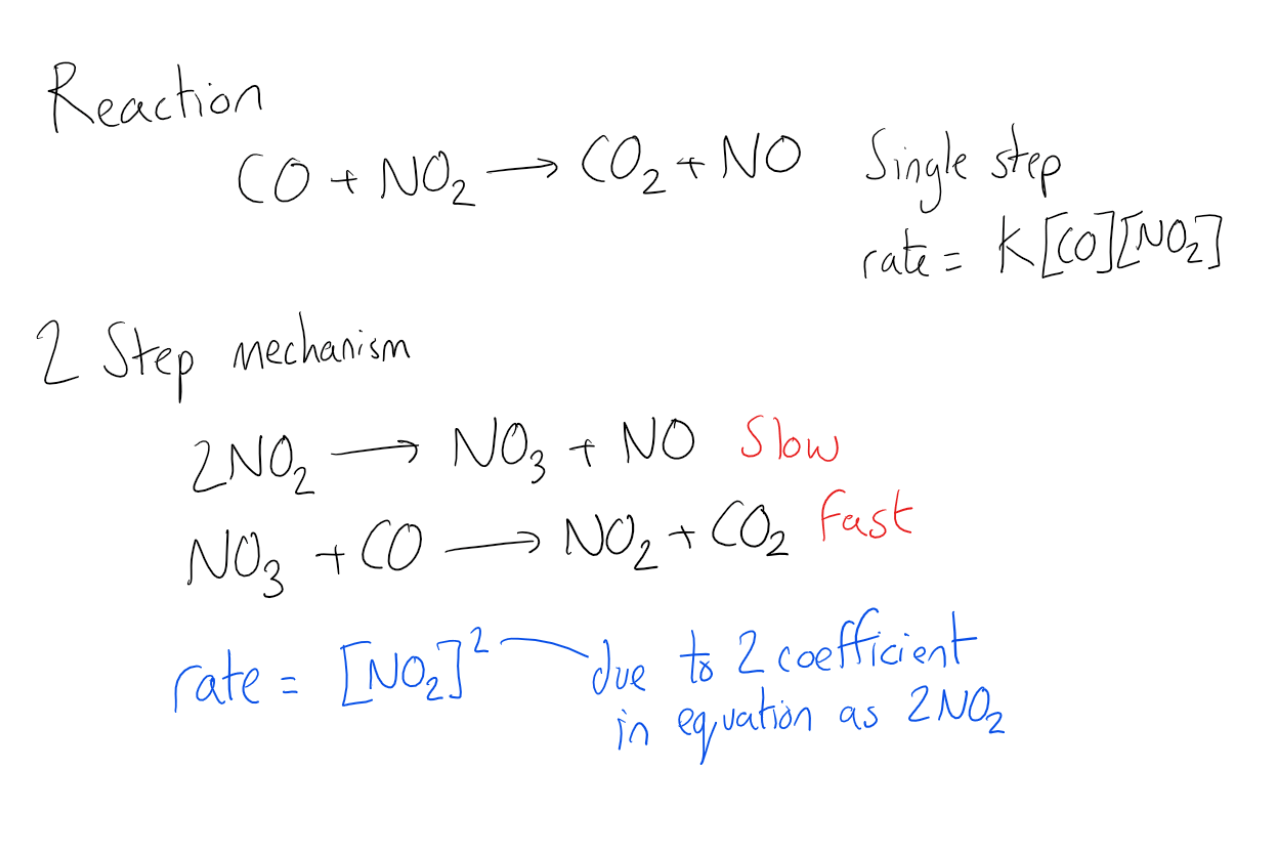Complimentary 1-hour tutoring consultation

### Rate-Determining Step

Topic: Rate Processes In Chemical Reactions Kinetics And Equilibrium

The rate of a multi-step reaction is determined by the slowest elementary step, which is known as the rate-determining step.

The determination of a reaction mechanism can only be made in the laboratory. When a reaction occurs in a sequence of elementary steps, the overall reaction rate is governed by whichever one of those steps is the slowest. The rate-determining step is the slowest step in the sequence of steps in a reaction mechanism.

Chemists often write chemical equations for reactions as a single step, which shows only a reaction’s net result. However, most chemical reactions occur over a series of elementary reactions. The reaction mechanism is the step-by-step process by which reactants actually become products. The overall reaction rate depends almost entirely on the rate of the slowest step. If the first step is the slowest, and the entire reaction must wait for it, then it is the rate-determining step.

For example, in the reaction of carbon monoxide and nitrogen dioxide to form carbon dioxide and nitrogen oxide the rate equation would usually be r=k[NO2][CO] as the rate depends on the reaction of the nitrogen dioxide and carbon monoxide in a one-step reaction. But if this was a multistep reaction and it was actually dependent on the collision of two nitrogen dioxide molecules the rate of reaction would be r = k[NO2]2. The fact that the experimentally-determined rate law does not match the rate law derived from the overall reaction equation suggests that the reaction occurs over multiple steps. Further, the experimental rate law is second-order, suggesting that the reaction rate is determined by a step in which two NO2 molecules react, and therefore the CO molecule must enter at another, faster step. Since the first step is the slowest, and the entire reaction must wait for it, it is the rate-determining step.Key Points

• Most reaction mechanisms are step-by-step processes by which reactants actually become products.

• The overall reaction rate depends almost entirely on the rate of the slowest step. If the first step is the slowest, and the entire reaction must wait for it, then it is the rate-determining step.

Key Terms

Reaction rate: How fast or slowly a reaction takes place.

Rate-determining step: The slowest individual transformation in a reaction mechanism.

Billing Information